# Spooky action at a distance in the loss landscape

Not all global minima of the (training) loss landscape are created equal.

Even if they achieve equal performance on the training set, different solutions can perform very differently on the test set or out-of-distribution. So why is it that we typically find "simple" solutions that generalize well?

In a previous post, I argued that the answer is "singularities" — minimum loss points with ill-defined tangents. It's the "nastiest" singularities that have the most outsized effect on learning and generalization in the limit of large data. These act as implicit regularizers that lower the effective dimensionality of the model.

Even after writing this introduction to "singular learning theory", I still find this claim weird and counterintuitive. How is it that the local geometry of a few isolated points determines the global expected behavior over all learning machines on the loss landscape? What explains the "spooky action at a distance" of singularities in the loss landscape?

Today, I'd like to share my best efforts at the hand-waving physics-y intuition behind this claim.

It boils down to this: singularities translate random motion at the bottom of loss basins into search for generalization.

# Random walks on the minimum-loss sets

Let's first look at the limit in which you've trained so long that we can treat the model as restricted to a set of fixed minimum loss points1.

Here's the intuition pump: suppose you are a random walker living on some curve that has singularities (self-intersections, cusps, and the like). Every timestep, you take a step of a uniform length in a random available direction. Then, singularities act as a kind of "trap." If you're close to a singularity, you're more likely to take a step towards (and over) the singularity than to take a step away from the singularity.

It's not quite an attractor (we're in a stochastic setting, where you can and will still break away every so often), but it's sticky enough that the "biggest" singularity will dominate your stable distribution.

In the discrete case, this is just the well-known phenomenon of high-degree nodes dominating most of expected behavior of your graph. In business, it's behind the reason that Google exists. In social networks, it's similar to how your average friend has more friends than you do.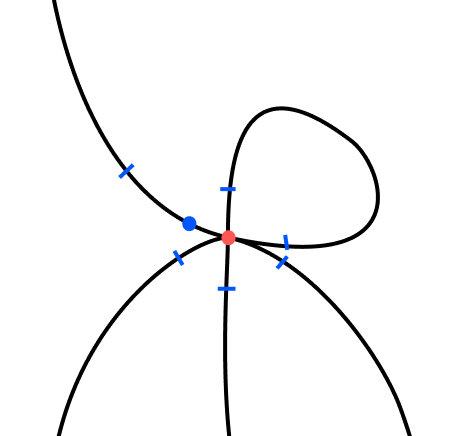To see this, consider a simple toy example: take two polygons and let them intersect at a single point. Next, let a random walker run loose on this setup. How frequently will the random walker cross each point?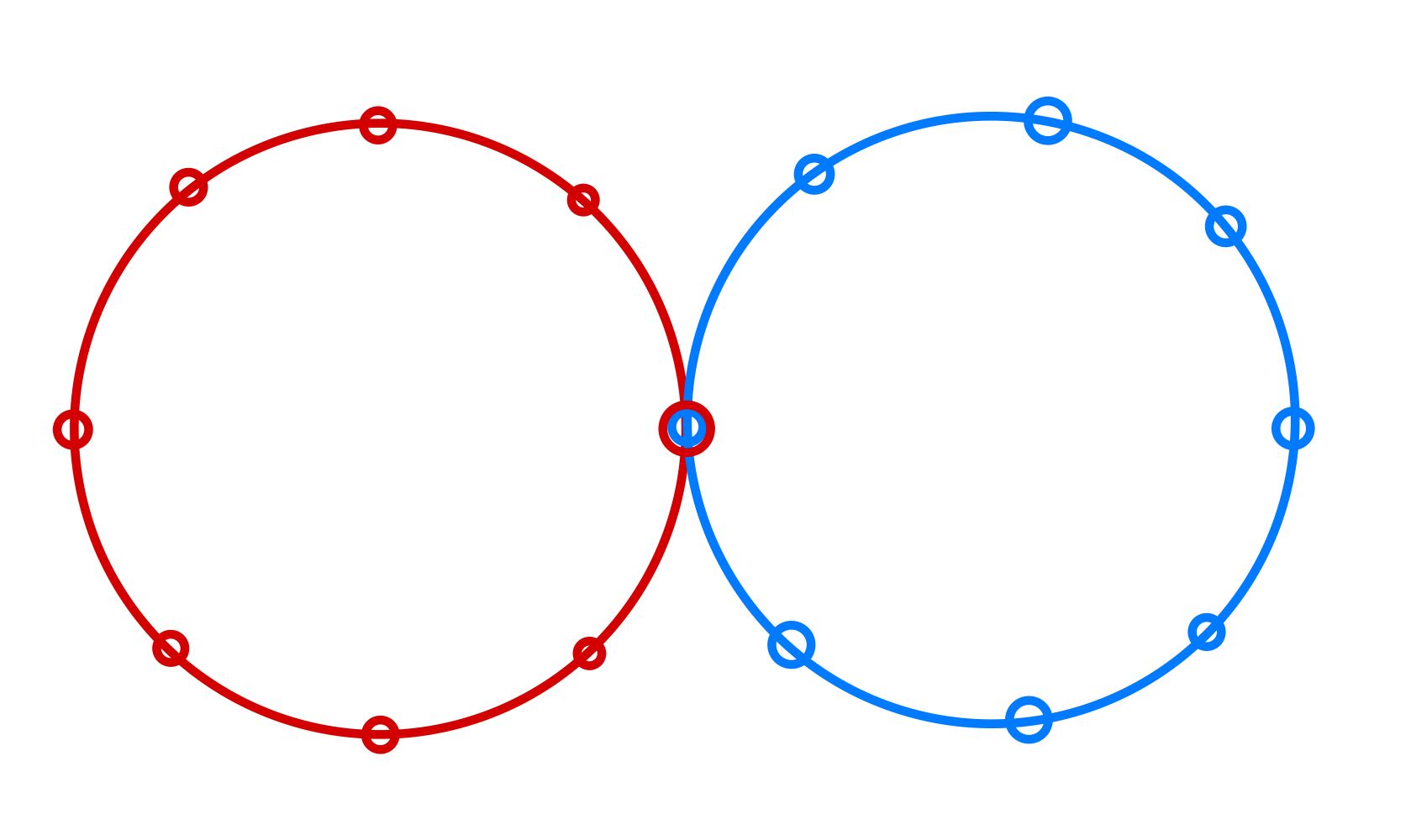Take two or more 1D lattices with toroidal boundary conditions and let them intersect at one point. In the limit of an infinite polygon/lattice, you end up with a normal crossing singularity at the origin.

If you've taken a course in graph theory, you may remember that the stable distribution weights nodes in proportion to their degrees. For two intersecting lines, the origin is twice as likely as the other points. For three intersecting lines, it's three times as likely, and so on…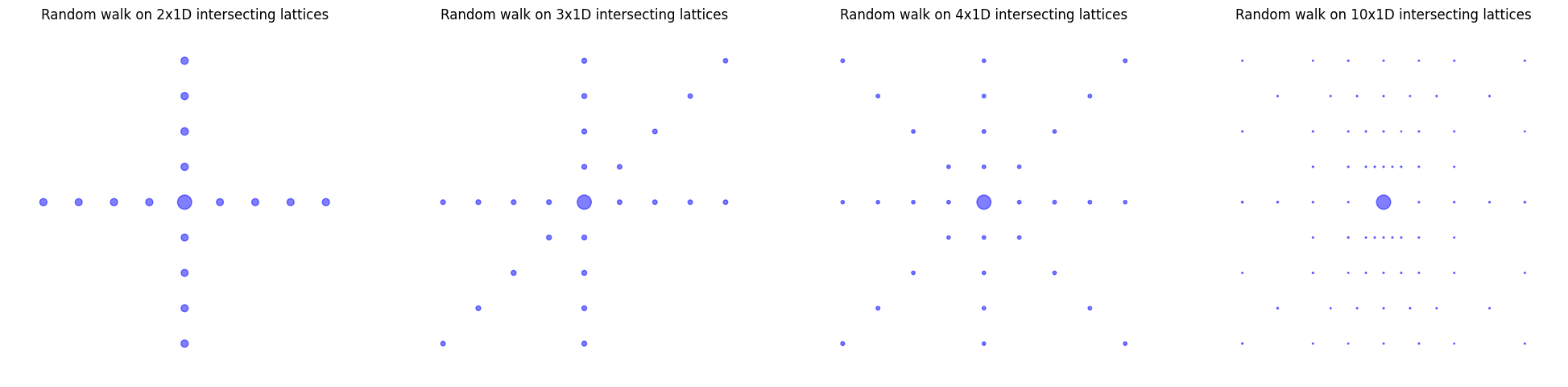The size of the circle shows how likely that point is under empirical simulation. The stationary distribution puts as many times more weight on the origin as there are intersecting lines.

Now just take the limit of infinitely large polygons/step size to zero, and we'll recover the continuous case we were originally interested in.

# Brownian motion near the minimum-loss set

Well, not quite. You see, restricting ourselves to motion along the minimum-loss points is unrealistic. We're more interested in messy reality, where we're allowed some freedom to bounce around the bottoms of loss basins.2

This time around, the key intuition-pumping assumption is to view the limiting behavior of stochastic gradient descent as a kind of Brownian motion — a source of randomness that no longer substantially improves loss but just jiggles us between solutions that are equivalent from the perspective of the training set.

To understand these dynamics, we can just study the more abstract case of Brownian motion in some continuous energy landscape with singularities.

Consider the potential function given by

$U(\boldsymbol x) = a \cdot \min((x_0-b)^2, (x_1-b)^2).$

This is plotted on the left side of the following figure. The right side depicts the corresponding stable distribution on the right as predicted by "regular" physics.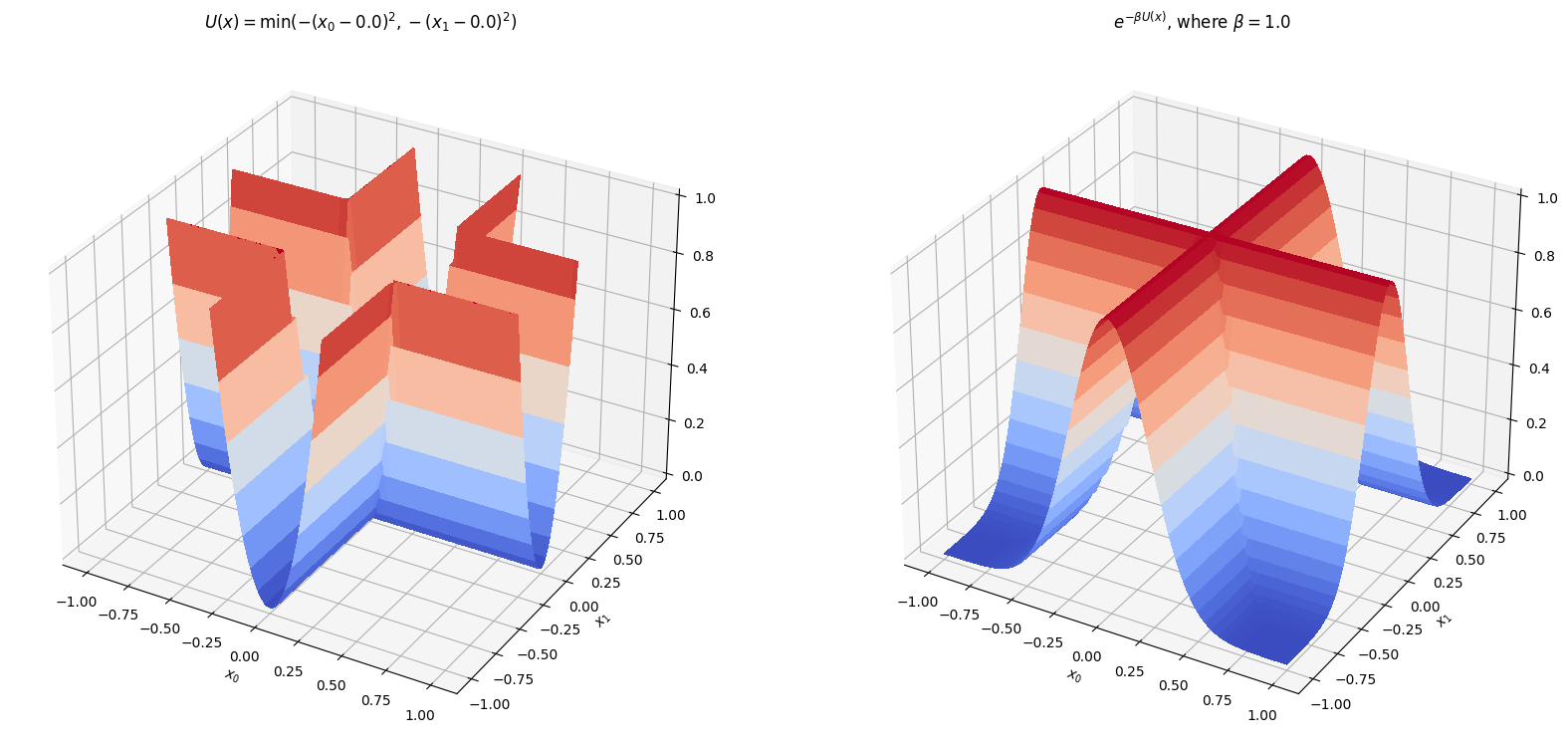An energy landscape whose minimum loss set has a normal crossing singularity at the origin. Toroidal boundary conditions as in the discrete case.

Simulating Brownian motion in this well generates an empirical distribution that looks rather different from the regular prediction…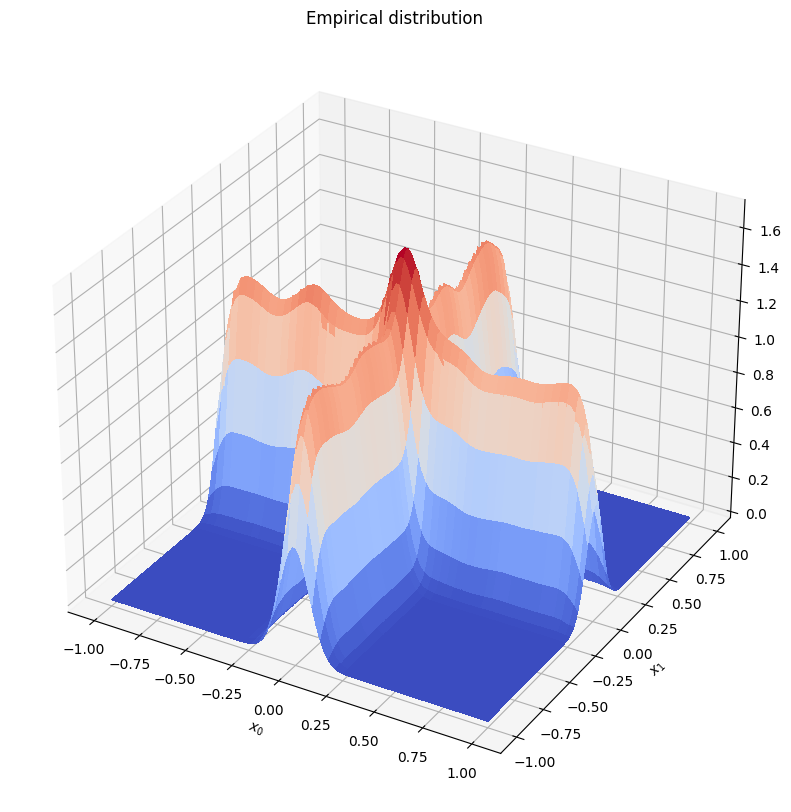As in the discrete case, the singularity at the origin gobbles up probability mass, even at finite temperatures and even for points away from the minimum loss set.

## Takeaways

To summarize, the intuition3 is something like this: in the limiting case, we don't expect the model to learn much from any one additional sample. Instead, the randomness in drawing the new sample acts as Brownian motion that lets the model explore the minimum-loss set. Singularities are a trap for this Brownian motion which allow the model to find well-generalizing solutions just by moving around.

In short, singularities work because they transform random motion into useful search for generalization.

You can find the code for these simulations here and here.

## Footnotes

1. So technically, in singular learning theory we treat the loss landscape as changing with each additional sample. Here, we're considering the case that the landscape is frozen, and new samples act as a kind of random motion along the minimum-loss set.

2. We're still treating the loss landscape as frozen but will now allow departures away from the minimum loss points.

3. Let me emphasize: this is hand-waving/qualitative/physics-y jabber. Don't take it too seriously.

# How to Perturb Weights

I'm running a series of experiments that involve some variation of: (1) perturb a weight initialization; (2) train the perturbed and baseline models in parallel, and (3) track how the perturbation grows/shrinks over time.

Naively, if we're interested in a perturbation analysis of the choice of weight initialization, we prepare some baseline initialization, $\mathbf w_0$, and then apply i.i.d. Gaussian noise, $\boldsymbol \delta$, to each of its elements, $\delta_i \sim \mathcal N(0, \epsilon^2)$. (If we want, we can let this vary layer-by-layer and let it depend on, for example, the norm of the layer it's being applied to.)

The problem with this strategy is that the perturbed weights $\mathbf w = \mathbf w_0 + \boldsymbol\delta$ are, in general, no longer sampled from the same distribution as the baseline weights.

There is nothing wrong with this per se, but it introduces a possible confounder (the thickness). This is especially relevant if we're interested specifically in the question of how behavior changes with the size of a perturbation, this problem introduces a possible confounder. As responsible experimentalists, we don't like confounders.

Fortunately, there's an easy way to "clean up" Kaiming He to make it better suited to this perturbative analysis.

# Kaiming initialization lives in a hyperspherical shell

Consider a matrix, $\mathbf w^{(l)}$, representing the weights of a particular layer $l$ with shape $(D_\mathrm{in}^{(l)}, D_\mathrm{out}^{(l+1)})$. $D_\mathrm{in}^{(l)}$ is also called the fan-in of the layer, and $D_\mathrm{in}^{(l+1)}$ the fan-out. For ease of presentation, we'll ignore the bias, though the following reasoning applies equally well to the bias.

We're interested in the vectorized form of this matrix, $\vec w^{(l)} \in \mathbb R^{D^{(l)}}$, where $D^{(l)} =D_\mathrm{in}^{(l)} \times D_\mathrm{out}^{(l+1)}$.

In Kaiming initialization, we sample the components, $w_i^{(l)}$, of this vector, i.i.d. from a normal distribution with mean 0 and variance $\sigma^2$ (where $\sigma^2 = \frac{2}{D_\mathrm{in}^{(l)}}$).

Geometrically, this is equivalent to sampling from a hyperspherical shell, $S^{D-1}$ with radius $\sqrt{D}\sigma$ and (fuzzy) thickness, $\delta$. (Ok, so technically, because the radius can vary from layer-to-layer, it's a hyperellipsoidal shell.)

This follows from some straightforward algebra (dropping the superscript $l$ for simplicity):

$\mathbb E[|\mathbf w|^2] = \mathbb E\left[\sum_{i=1}^D w_i^2\right] = \sum_{i=1}^D \mathbb E[w_i^2] = \sum_{i=1}^D \sigma^2 = D\sigma^2,$

and

\begin{align} \delta^2 \propto \mathrm{var} [|\mathbf w|^2] &= \mathbb E\left[\left(\sum_{i=1}^D w_i^2\right)^2\right] - \mathbb E\left[\sum_{i=1}^D w_i^2\right]^2 \\ &= \sum_{i, j=1}^D \mathbb E[w_i^2 w_j^2] - (D\sigma^2)^2 \\ &= \sum_{i \neq j}^D \mathbb E[w_i^2] \mathbb E[w_j^2] + \sum_{i=1}^D \mathbb E[w_i^4]- (D\sigma^2)^2 \\ &= D(D-1) \sigma^4 + D(3\sigma^4) - (D\sigma^2)^2 \\ &= 2D\sigma^4. \end{align}

So the thickness as a fraction of the radius is

$\frac{\delta}{\sqrt{D}\sigma} = \frac{\sqrt{2D}\sigma}{\sqrt{D}} = \sqrt{2}\sigma = \frac{2}{\sqrt{D_\mathrm{in}^{(l)}}},$

where the last equality follows from the choice of $\sigma$ for Kaiming initialization.

This means that for suitably wide networks ($D_\mathrm{in}^{(l)} \to \infty$), the thickness of this shell goes to $0$.

# Taking the thickness to 0

This suggests an alternative initialization strategy. What if we immediately take the limit $D_\text{in}^{(l)} \to \infty$, and sample directly from the boundary of a hypersphere with radius $\sqrt{D}\sigma$, i.e., modify the shell thickness to be $0$.

This can easily be done by sampling each component from a normal distribution with mean 0 and variance $1$ and then normalizing the resulting vector to have length $\sqrt{D}\sigma$ (this is known as the Muller method).

# Perturbing Weight initialization

The modification we made to Kaiming initialization was to sample directly from the boundary of a hypersphere, rather than from a hyperspherical shell. This is a more natural choice when conducting a perturbation analysis, because it makes it easier to ensure that the perturbed weights are sampled from the same distribution as the baseline weights.

Geometrically, the intersection of a hypersphere $S^D$ of radius $w_0=|\mathbf w_0|$ with a hypersphere $S^D$ of radius $\epsilon$ that is centered at some point on the boundary of the first hypersphere, is a lower-dimensional hypersphere $S^{D-1}$ of a modified radius $\epsilon'$. If we sample uniformly from this lower-dimensional hypersphere, then the resulting points will follow the same distribution over the original hypersphere.

This suggests a procedure to sample from the intersection of the weight initialization hypersphere and the perturbation hypersphere.

First, we sample from a hypersphere of dimension $D-1$ and radius $\epsilon'$ (using the same technique we used to sample the baseline weights). From a bit of trigonometry, see figure below, we know that the radius of this hypersphere will be $\epsilon' = w_0\cos \theta$, where $\theta = \cos^{-1}\left(1-\frac{\epsilon^2}{2w_0^2}\right)$.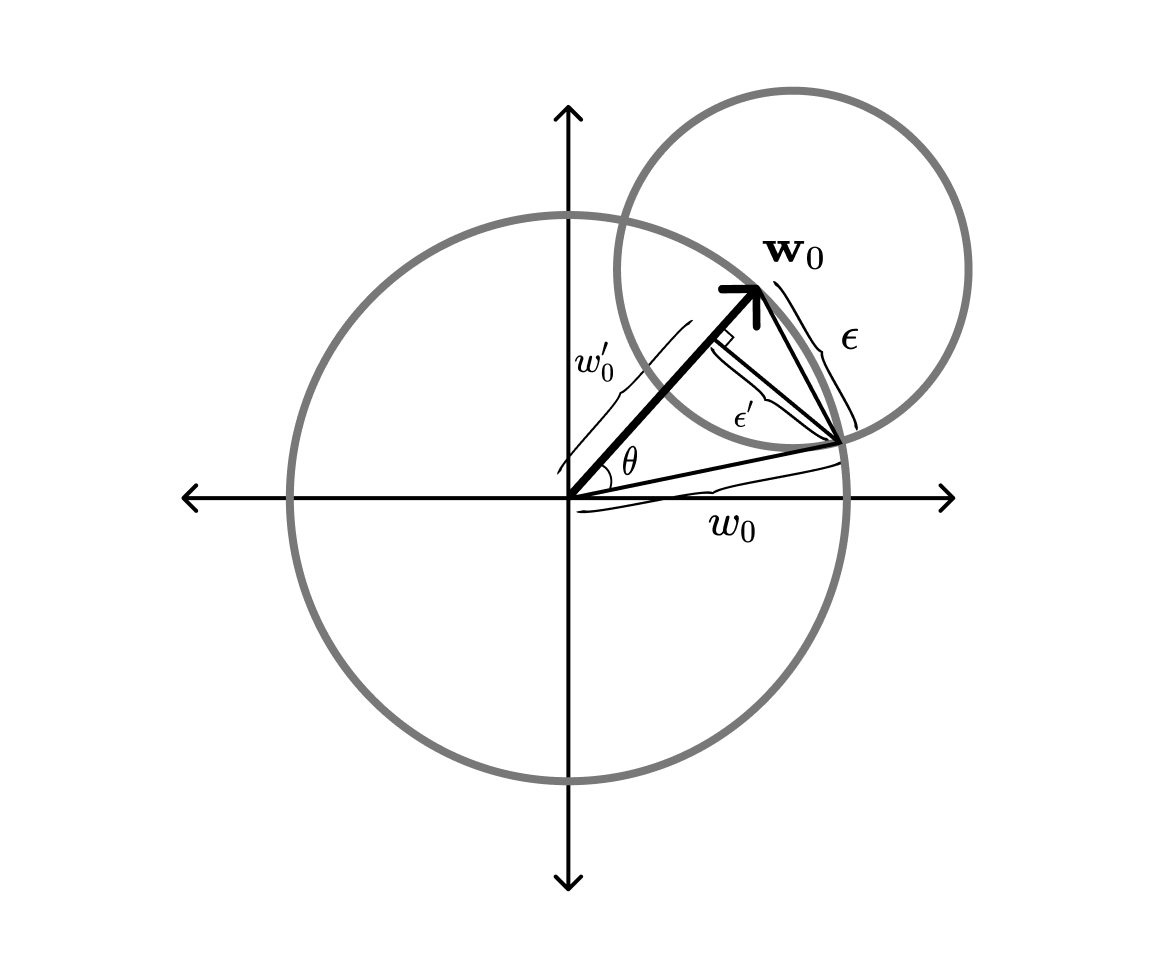Next, we rotate the vector so it is orthogonal to the baseline vector $\mathbf w_0$. This is done with a Householder reflection, $H$, that maps the current normal vector $\hat{\mathbf n} = (0, \dots, 0, 1)$ onto $\mathbf w_0$:

$H = \mathbf I - 2\frac{\mathbf c \mathbf c^T}{\mathbf c^T \mathbf c},$

where

$\mathbf c = \hat{\mathbf n} + \hat {\mathbf w}_0,$

and $\hat{\mathbf w}_0 = \frac{\mathbf w_0}{|w_0|}$ is the unit vector in the direction of the baseline weights.

Implementation note: For the sake of tractability, we directly apply the reflection via:

$H\mathbf y = \mathbf y - 2 \frac{\mathbf c^T \mathbf y}{\mathbf c^T\mathbf c} \mathbf c.$

Finally, we translate the rotated intersection sphere along the baseline vector, so that its boundary goes through the intersection of the two hyperspheres. From the figure above, we find that the translation has the magnitude $w_0' = w_0 \cos \theta$.

By the uniform sampling of the intersection sphere and the uniform sampling of the baseline vector, we know that the resulting perturbed vector will have the same distribution as the baseline vector, when restricted to the intersection sphere.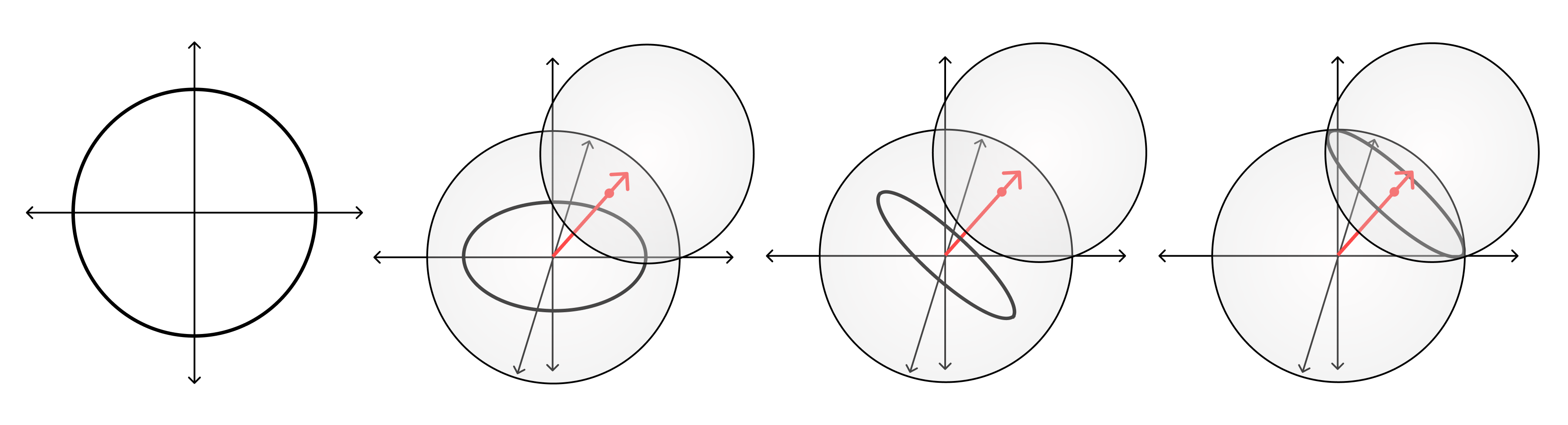# Random Walks on Hyperspheres

Neural networks begin their lives on a hypersphere.

As it turns out, they live out the remainder of their lives on hyperspheres as well. Take the norm of the vectorized weights, $\mathbf w$, and plot it as a function of the number of training steps. Across many different choices of weight initialization, this norm will grow (or shrink, depending on weight decay) remarkably consistently.

In the following figure, I've plotted this behavior for 10 independent initializations across 70 epochs of training a simple MNIST classifier. The same results hold more generally for a wide range of hyperparameters and architectures.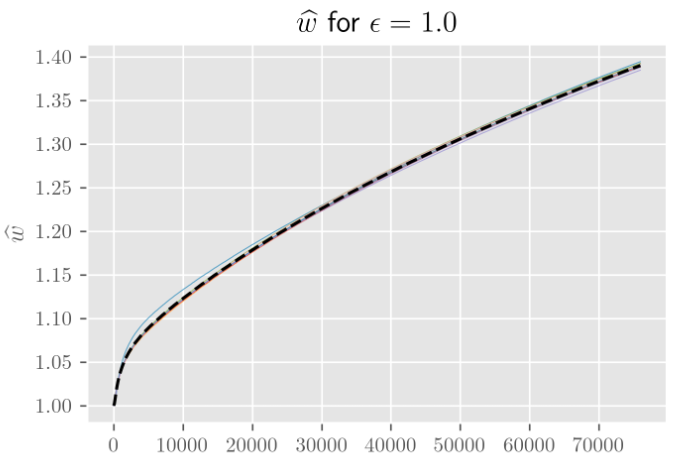The norm during training, divided by the norm upon weight initialization. The parameter ϵ controls how far apart (as a fraction of the initial weight norm) the different weight initializations are.

All this was making me think that I disregarded Brownian motion as a model of SGD dynamics a little too quickly. Yes, on lattices/planes, increasing the number of dimensions makes no difference to the overall behavior (beyond decreasing the variance in how displacement grows with time). But maybe that was just the wrong model. Maybe Brownian motion on hyperspheres is considerably different and is actually the thing I should have been looking at.

# Asymptotics ($t, n \to \infty$)

We'd like to calculate the expected displacement (=distance from our starting point) as a function of time. We're interested in both distance on the sphere and distance in the embedding space.

Before we tackle this in full, let's try to understand Brownian motion on hyperspheres in a few simplifying limits.

Unlike the Euclidean case, where having more than one dimension means you'll never return to the origin, living on a finite sphere means we'll visit every point infinitely many times. The stationary distribution is the uniform distribution over the sphere, which we'll denote $\mathcal U^{(n)}$ for $S^n$.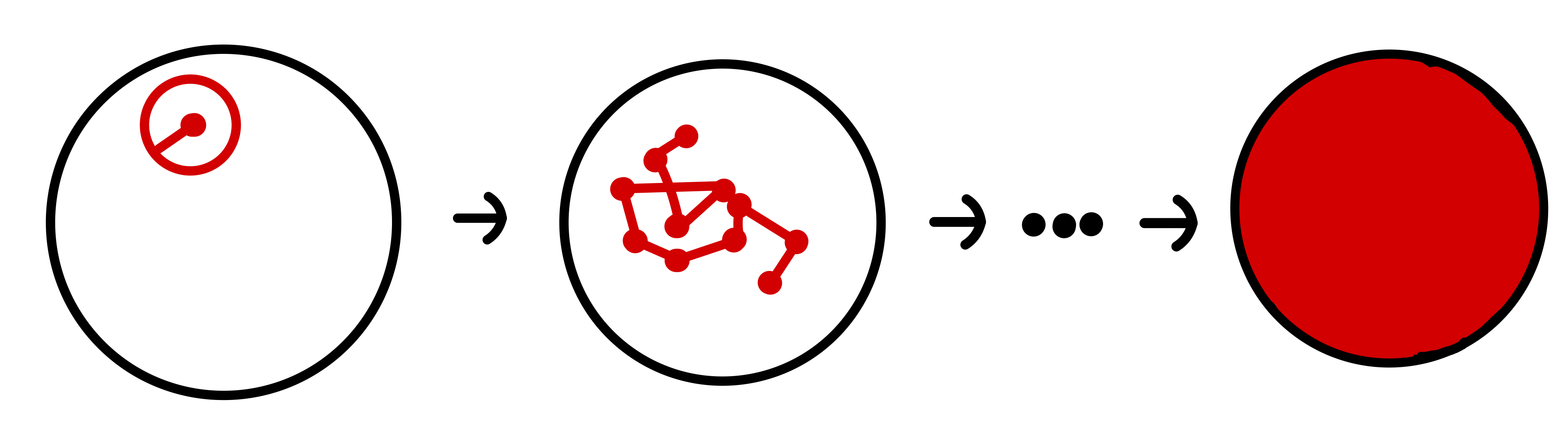So our expectations end up being relatively simple integrals over the sphere.

The distance from the pole at the bottom to any other point on the surface depends only on the angle from the line intersecting the origin and the pole to the line intersecting the origin and the other point,

$z(\theta) = \sqrt{(r\sin \theta)^2 - (r-r\cos \theta)^2} = 2r\sin \left(\frac{\theta}{2}\right).$

This symmetry means we only have to consider a single integral from the bottom to to the top of $S^{n-1}$ "slices." Each slice has as much probability associated to it as the corresponding $S^{n-1}$ has surface area times the measure associated to $\theta$ ($=r\ \mathrm d \theta$). This surface area varies with the angle from the center, as does the distance of that point from the origin. Put it together and you get some nasty integrals.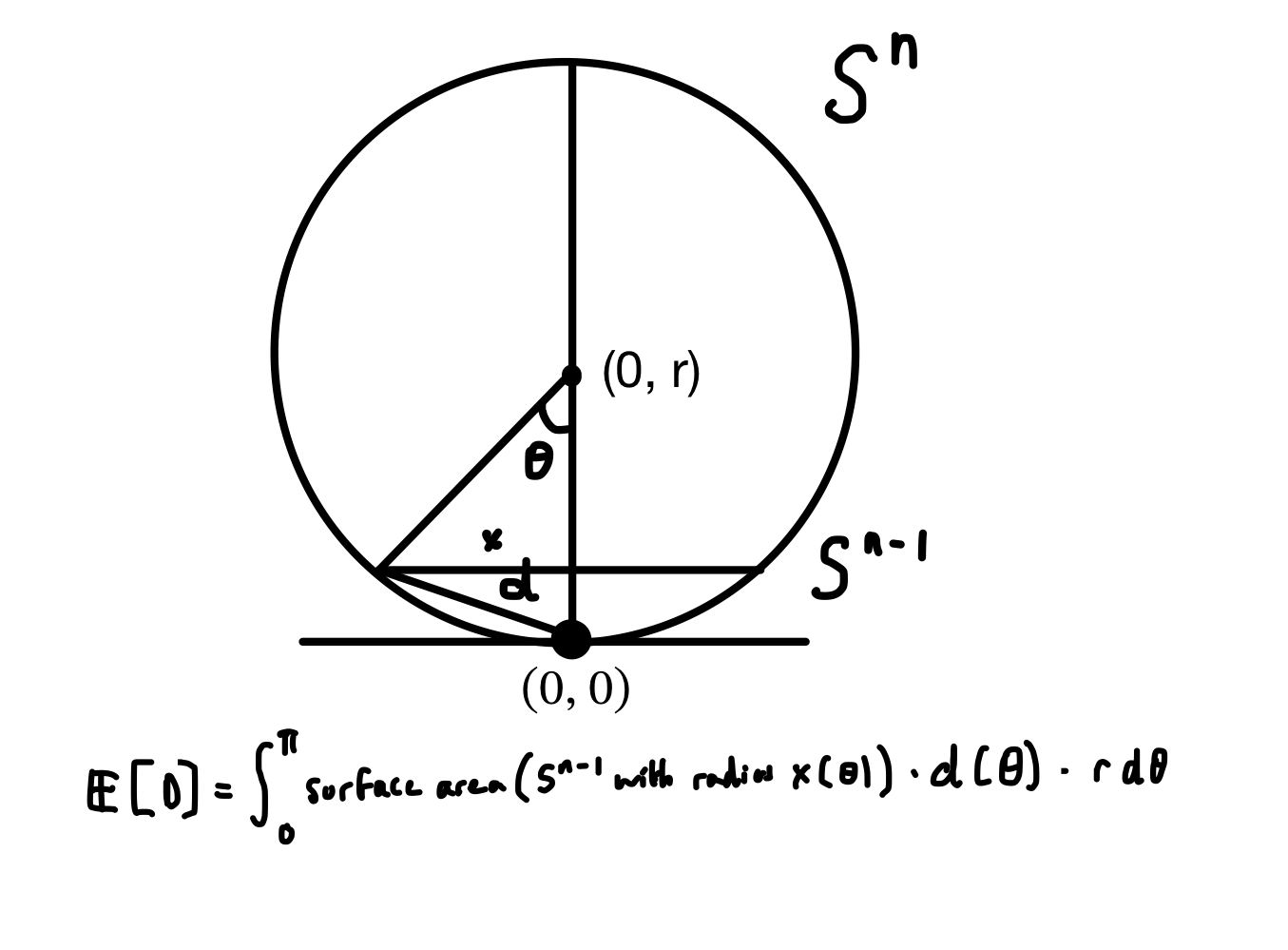From this point on, I believe I made a mistake somewhere (the analysis for $n \to \infty$ is correct).

If we let $S_{n}(x)$ denote the surface area of $S^{n}$ with radius $r$, we can fill in the formula from Wikipedia:

$S_{n-1}(x) = \frac{2\pi^{\frac{n}{2}}}{\Gamma(\frac{n}{2})} x^{n-1}.$

Filling in $x(\theta) =r \sin\theta$, we get an expression for the surface area of each slice as a function of $\theta$,

$S_{n-1}(x(\theta)) = S_{n-1}(r) \sin^{n-1}(\theta),$

so the measure associated to each slice is

$\mathrm d\mu(\theta) = S_{n-1}(r)\sin^{n-1}(\theta)\ r\, \mathrm d\theta.$

## $n \to \infty$

We can simplify further, as $n\to\infty$, the $\sin^{n-1}(\theta)$ will tend towards a "comb function," that is zero everywhere except for $\theta = (m + 1/2)\pi$ for integer $m$, where it is equal to $1$ if $m$ is even, and $-1$ if $m$ is odd.

Within the above integration limits $(0, \pi)$, this means all of the probability becomes concentrated at the middle, where $\theta=\pi/2$.

So in the infinite-dimensional limit, the expected distance from the origin is the distance between a point on the middle and one of its poles, which is $\sqrt{2} r$.

## General case

An important lesson I've learned here is: "try more keyword variations in Google Scholar and Elicit before going off and solving these nasty integrals yourself because you will probably make a mistake somewhere and someone else has probably already done the thing you're trying to do."

Anyway, here's the thing where someone else has already done the thing I was trying to do.  gives us the following form for the expected cosine of the angular displacement with time:

$\langle\cos \theta(t)\rangle=\exp \left(-D(n-1) t / R^2\right).$

A little trig tells us that the expected displacement in the embedding space is

$\langle z(t)\rangle = r \sqrt{2\cdot(1-\langle \cos\theta\rangle)},$

which gives us a set of nice curves depending on the diffusion constant $D$: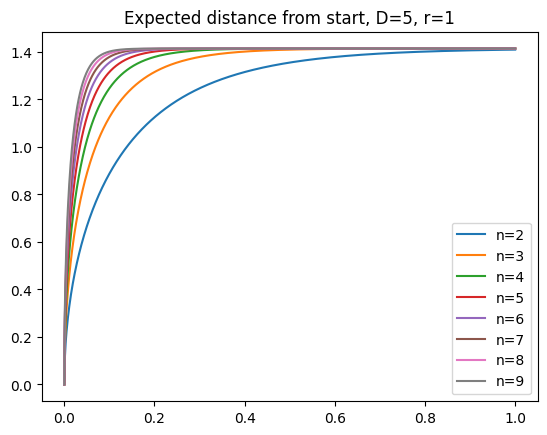The major difference with Brownian motion on the plane is that expected displacement asymptotes at the limit we argued for above ($\sqrt{2} r$).

When I had initially looked at curves like the following (this ones from [numerical simulation](https://github.com/jqhoogland/experiminis/blob/main/brownian_hyperspheres.ipynb, not the formula), I thought I had struck gold.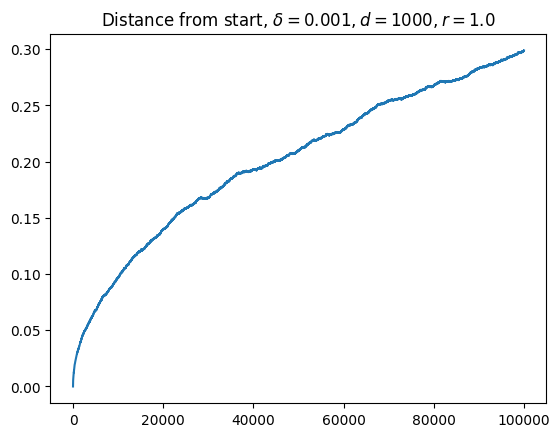I've been looking for a model that predicts square root like growth at the very start, followed by a long period of nearly linear growth, followed by asymptotic behavior. Eyeballing this, it looked like my searching for the long plateau of linear growth had come to an end.

Of course, this was entirely wishful thinking. Human eyes aren't great discriminators of lines. That "straight line" is actually pretty much parabolic. As I should have known (from the Euclidean nature of manifolds), the initial behavior is incredibly well modeled by a square root.

As you get further away from the nearly flat regime, the concavity only increases. This is not the curve I was looking for.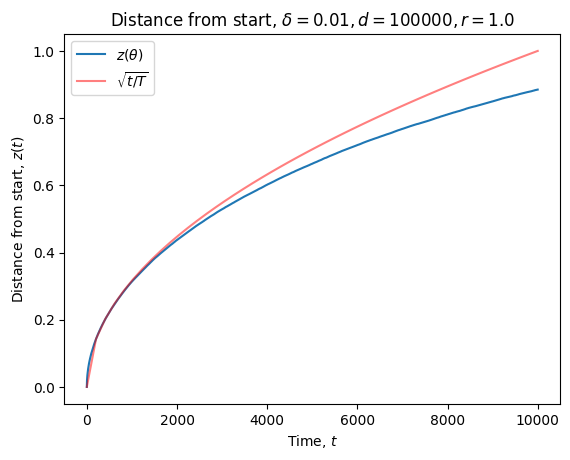So my search continues.

# Beyond Bayes

Context: We want to learn an appropriate function $f$ provided samples from a dataset $D_n = \{(X, Y)\}^n$.

Turns out, you can do better than the naive Bayes update,

$P(f|D_n) = \frac{P(D_n| f)\ P(f)}{P(D_n)}.$

# Tempered Bayes

Introduce an inverse temperature, $\beta$, to get the tempered Bayes update :

$P_\beta(f|D_n) = \frac{P(D_n|f)^{\beta}\ P(f)}{P_{\beta}(D_n)}.$

At first glance, this looks unphysical. Surely $P(A|B)^{\beta}\ P(B) = P(A, B)$ only when $\beta=1$?

If you're one for handwaving, you might just accept that this is just a convenient way to vary between putting more weight on the prior and more weight on the data. In any case, the tempered posterior is proper (integrable to one), as long as the untempered posterior is .

If you're feeling more thorough, think about the information. Introducing an inverse temperature is simply scaling the number of bits contained in the distribution. $P(X, Y|f) = \exp\{-\beta I(X, Y|f)\}$.

TODO: Check out Grünwald's Safe Bayes papers

# Generalized Bayes

If you're feeling even bolder, you might replace the likelihood with a general loss term, $\ell_{\beta, n}(f)$, which measures performance on your dataset $D_n$,

$P_\beta(f|D_{n)}= \frac{\ell_{\beta,n}(f)\ P(f)}{Z_n},$

where we write the normalizing constant or partition function as $Z_n$ to emphasize that it isn't really an "evidence" anymore.

The most natural choice for $\ell_{\beta,n}$ is the Gibbs measure:

$\ell_{\beta, n}(f) = \exp\left\{-\beta\, r_n(f)\right\},$

where $r_n$ is the empirical risk of classical machine learning. You can turn any function into a probability.

TODO

# You can turn any function into a probability

Machine learning has gotten sloppy over the years.

It used to be that we thought carefully about the theoretical underpinnings of our models and proceeded accordingly.

We used the L2 loss in regression because, when doing maximum likelihood estimation over, L2 loss follows from the assumption that our samples are distributed according to i.i.d. Gaussian noise around some underlying deterministic function, $f_w$. If we have a likelihood defined as

\begin{align} p(D_n|w) = \prod_{i=1}^np(x_{i}, y_{i}|w),\quad&\text{where}\quad p(x_{i}, y_{i}) \sim \mathcal N(y_{i}|f_{w}(x_{i}), \sigma^2),\\ \implies\underset{x}{\text{argmax}}\ p(D_n|w)&=\underset{x}{\text{argmax}}\ \sum\limits_{i=1}^{n} (y_{i} - f_w(x_i))^2, \end{align}

where $w$ are the weights parametrizing our model.

We'd squeeze in some weight decay because when performing maximum a posteriori estimation it was equivalent to having a Gaussian prior over our weights, $\varphi(w)\sim \mathcal N(0, \lambda^{-1})$. For the same likelihood as above,

$\underset{x}{\text{argmax}}\ p(D_n|w)\varphi(w) = \underset{x}{\text{argmax}}\ \left(\sum\limits_{i=1}^{n}(y_i-f_w(x_{i}))^{2}\right)- \lambda |w|^2.$

Nowadays, you just choose a loss function and twiddle with the settings until it works. Granted, this shift away from Bayesian-grounded techniques has given us a lot of flexibility. And it actually works (unlike much of the Bayesian project which turns out to just be disgustingly intractable).

But when you're a theorist trying to catch up to the empirical results, it turns out the Bayesian frame is rather useful. So we want a principled way of recovering probability distributions from arbitrary choices of loss function. Fortunately, this is possible.

The trick is simple: multiply your loss, $r_n(w)$, by some parameter $\beta$, whose units are such that $\beta\, r_n(w)$ is measured in bits. Now, negate and exponentiate and out pop a set of probabilities:

$p(D_n|w) = \frac{e^{-\beta\, r_{n}({w})}}{Z_n}.$

We put a probability distribution over the function space we want to model, and we can extract probabilities over outputs for given inputs.